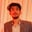Trusted answers to developer questions
Trusted Answers to Developer Questions

Related Tags

project euler
46
communitycreator

# What is Project Euler 46?Armaan Nougai

Grokking Modern System Design Interview for Engineers & Managers

Ace your System Design Interview and take your career to the next level. Learn to handle the design of applications like Netflix, Quora, Facebook, Uber, and many more in a 45-min interview. Learn the RESHADED framework for architecting web-scale applications by determining requirements, constraints, and assumptions before diving into a step-by-step design process.### Problem statement

Project Euler 46 is Goldbach’s other conjecture. He proposed that every odd number can be written as a sum of a prime and twice a square, i.e., for any odd number, the following is true:

N = p + 2*(i)

Where p is a prime number, and i is a perfect square. It turns out this conjecture is false.

So, what is the smallest odd composite number that cannot be written as the sum of a prime and twice a square?

### Solution approach

Out of various brute force solutions that are applicable here, one of the best and most efficient brute force solutions is as follows:

1. We will run a while loop on every odd composite number (n).
2. For every n, we will generate all primes (p) less than n.
3. Then for every p, we will calculate i (i=(n-p)/2) and check if i is a perfect square.
4. We will then break the loop if, for a particular n, we find no (p,i) satisfying n=p+2*i.

### Solution implementation

#### The following two functions are imported:

1. math.sqrt(n) returns the square root of a number n.

2. bisect.bisect(A,x) returns the position in which x should be inserted so that after insertion, the already sorted array A remains sorted. In simpler words, it returns an index of the first element greater than x in a sorted array A.

#### The other two functions are:

1. gen_prime(n) generates all prime numbers between the last element of primes and n.
2. check(term) checks if there exists any (p,i) pair for a particular n.
"""Coded by - Armaan Nougai"""from math import sqrtfrom bisect import bisectprimes = [2,3,5]def gen_prime(n):    #generating primes less than n.    global primes    for possiblePrime in range(primes[-1],n+1,2):           i=0        while i<bisect(primes,possiblePrime//(i+1)):            if possiblePrime%primes[i] == 0:                break            i+=1        else:            primes += [possiblePrime]def check(n):    # checking if there exist (p,i) pair    for p in primes[:bisect(primes,n)]:        i=(n-p)//2        if int(sqrt(i))**2==i:            # (p,i) pair found            return True    # no (p,i) pair found    return False    num = 3while True:    gen_prime(num)    if num not in primes and not check(num) :        print(num)        break    num += 2

RELATED TAGS

project euler
46
communitycreator

CONTRIBUTORArmaan Nougai

Grokking Modern System Design Interview for Engineers & Managers

Ace your System Design Interview and take your career to the next level. Learn to handle the design of applications like Netflix, Quora, Facebook, Uber, and many more in a 45-min interview. Learn the RESHADED framework for architecting web-scale applications by determining requirements, constraints, and assumptions before diving into a step-by-step design process.Keep Exploring

Learn in-demand tech skills in half the time